### 家常菜### var r2 = '《我爱每一天》 20110819 涛式私房菜'; if (r2.length>=25) { document.write(r2.substr(0,23)+'...') } else { document.write(r2); }### var r2 = '《我爱每一天》 20110824 神奇的紫苏'; if (r2.length>=25) { document.write(r2.substr(0,23)+'...') } else { document.write(r2); }### var r2 = '《我爱每一天》 20110818 腌瓜肉末'; if (r2.length>=25) { document.write(r2.substr(0,23)+'...') } else { document.write(r2); }### var r2 = '《我爱每一天》 20110817 西班牙口蘑牛肉'; if (r2.length>=25) { document.write(r2.substr(0,23)+'...') } else { document.write(r2); }### var r2 = '《我爱每一天》 20110902 乐活福星秀'; if (r2.length>=25) { document.write(r2.substr(0,23)+'...') } else { document.write(r2); }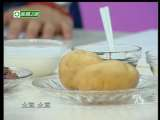### var r2 = '《美味人生》 20110817 于晓光 红酒牛排'; if (r2.length>=25) { document.write(r2.substr(0,23)+'...') } else { document.write(r2); }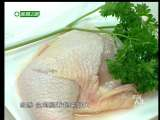### var r2 = '《美味人生》 20110816 鱼香煎鸡腿'; if (r2.length>=25) { document.write(r2.substr(0,23)+'...') } else { document.write(r2); }### 主食### var r2 = '《我爱每一天》 20110831 意式花样烤面包'; if (r2.length>=25) { document.write(r2.substr(0,23)+'...') } else { document.write(r2); }### var r2 = '《我爱每一天》 20110823 减肥甜品'; if (r2.length>=25) { document.write(r2.substr(0,23)+'...') } else { document.write(r2); }### var r2 = '《我爱每一天》 20110825 风车鸡肉芝士卷'; if (r2.length>=25) { document.write(r2.substr(0,23)+'...') } else { document.write(r2); }### var r2 = '《我爱每一天》 20110901 意大利蛋饼卷'; if (r2.length>=25) { document.write(r2.substr(0,23)+'...') } else { document.write(r2); }### var r2 = '《美味人生》 经典英式下午茶'; if (r2.length>=25) { document.write(r2.substr(0,23)+'...') } else { document.write(r2); }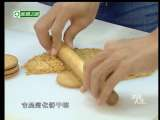### var r2 = '《美味人生》 20110815 鲜果柠檬芝士蛋糕'; if (r2.length>=25) { document.write(r2.substr(0,23)+'...') } else { document.write(r2); }### var r2 = '《美味人生》 20110824 意大利风味爱心蛋包饭 包住爱人的心'; if (r2.length>=25) { document.write(r2.substr(0,23)+'...') } else { document.write(r2); }### 汤羹### var r2 = '《我爱每一天》 20110819 涛式私房菜'; if (r2.length>=25) { document.write(r2.substr(0,23)+'...') } else { document.write(r2); }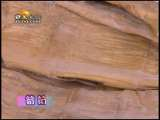### var r2 = '《美女私房菜》 筍筒腌肉豆腐汤 2011-02-27'; if (r2.length>=25) { document.write(r2.substr(0,23)+'...') } else { document.write(r2); }### var r2 = '美女私房菜 莲藕鸭汤 20100919'; if (r2.length>=25) { document.write(r2.substr(0,23)+'...') } else { document.write(r2); }### var r2 = '美女私房菜 2010-05-09'; if (r2.length>=25) { document.write(r2.substr(0,23)+'...') } else { document.write(r2); }### var r2 = '《美女私房菜》 沙参海参瘦肉汤 2011-04-30'; if (r2.length>=25) { document.write(r2.substr(0,23)+'...') } else { document.write(r2); }### var r2 = '家政女皇 懒人凉汤'; if (r2.length>=25) { document.write(r2.substr(0,23)+'...') } else { document.write(r2); }### var r2 = '家政女皇 酸辣汤'; if (r2.length>=25) { document.write(r2.substr(0,23)+'...') } else { document.write(r2); }### 地方小吃### var r2 = '[玩转美食]糖不甩'; if (r2.length>=25) { document.write(r2.substr(0,23)+'...') } else { document.write(r2); }### var r2 = '[玩转美食]酱爆烤鸭配老油条'; if (r2.length>=25) { document.write(r2.substr(0,23)+'...') } else { document.write(r2); }### var r2 = '[玩转美食]避风塘酿茄子'; if (r2.length>=25) { document.write(r2.substr(0,23)+'...') } else { document.write(r2); }### var r2 = '我在中国过大节湖南系列 第3集 湘潭古怪小年饭'; if (r2.length>=25) { document.write(r2.substr(0,23)+'...') } else { document.write(r2); }### var r2 = '我在中国过大节东北系列 第2集 岁末的集市狂欢'; if (r2.length>=25) { document.write(r2.substr(0,23)+'...') } else { document.write(r2); }### var r2 = '我在中国过大节东北系列 第1集 俺们村的杀猪菜'; if (r2.length>=25) { document.write(r2.substr(0,23)+'...') } else { document.write(r2); }### var r2 = '我在中国过大节湖南系列 第2集 鞭炮之乡喜事多'; if (r2.length>=25) { document.write(r2.substr(0,23)+'...') } else { document.write(r2); }### 厨房妙招### var r2 = '《我爱每一天》 20110816 健康吃水果'; if (r2.length>=25) { document.write(r2.substr(0,23)+'...') } else { document.write(r2); }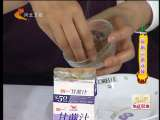### var r2 = '家政女皇 自制三层冰棍'; if (r2.length>=25) { document.write(r2.substr(0,23)+'...') } else { document.write(r2); }### var r2 = '家政女皇 10分钟做卤蛋'; if (r2.length>=25) { document.write(r2.substr(0,23)+'...') } else { document.write(r2); }### var r2 = '家政女皇 自制秋梨膏'; if (r2.length>=25) { document.write(r2.substr(0,23)+'...') } else { document.write(r2); }### var r2 = '家政女皇 蛋糕保鲜'; if (r2.length>=25) { document.write(r2.substr(0,23)+'...') } else { document.write(r2); }### var r2 = '家政女皇 巧去火锅沫'; if (r2.length>=25) { document.write(r2.substr(0,23)+'...') } else { document.write(r2); }### var r2 = '家政女皇 巧切土豆丝'; if (r2.length>=25) { document.write(r2.substr(0,23)+'...') } else { document.write(r2); }### 美食专题### 推荐给你一周的汤水### 美食论坛### 明星喜欢去哪里用餐### 美食博客